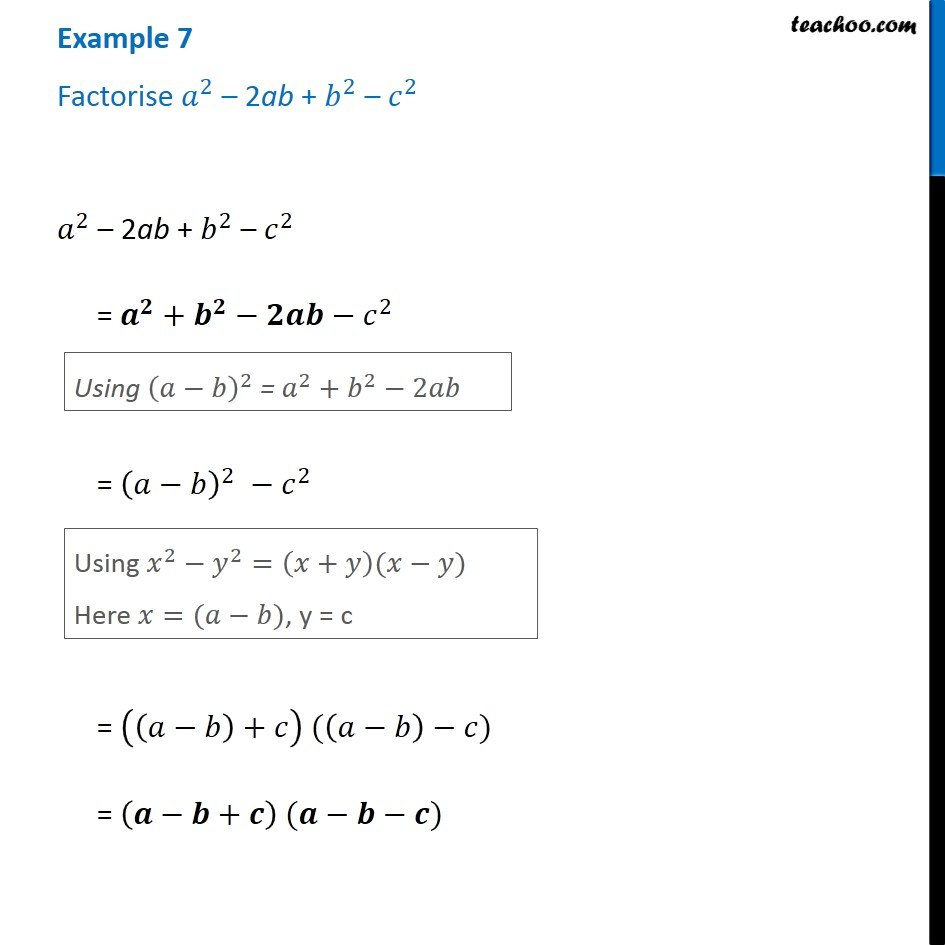Subscribe to our Youtube Channel - https://you.tube/teachoo

1. Chapter 14 Class 8 Factorisation
2. Serial order wise
3. Examples

Transcript

Example 7 Factorise 𝑎^2 – 2ab + 𝑏^2 – 𝑐^2 𝑎^2 – 2ab + 𝑏^2 – 𝑐^2 = 𝒂^𝟐+𝒃^𝟐−𝟐𝒂𝒃−𝑐^2 Using (𝑎−𝑏)^2 = 𝑎^2+𝑏^2−2𝑎𝑏 = (𝑎−𝑏)^2 −𝑐^2 Using 𝑥^2−𝑦^2=(𝑥+𝑦)(𝑥−𝑦) Here 𝑥=(𝑎−𝑏), y = c = ((𝑎−𝑏)+𝑐) ((𝑎−𝑏)−𝑐) = (𝒂−𝒃+𝒄) (𝒂−𝒃−𝒄)

Examples